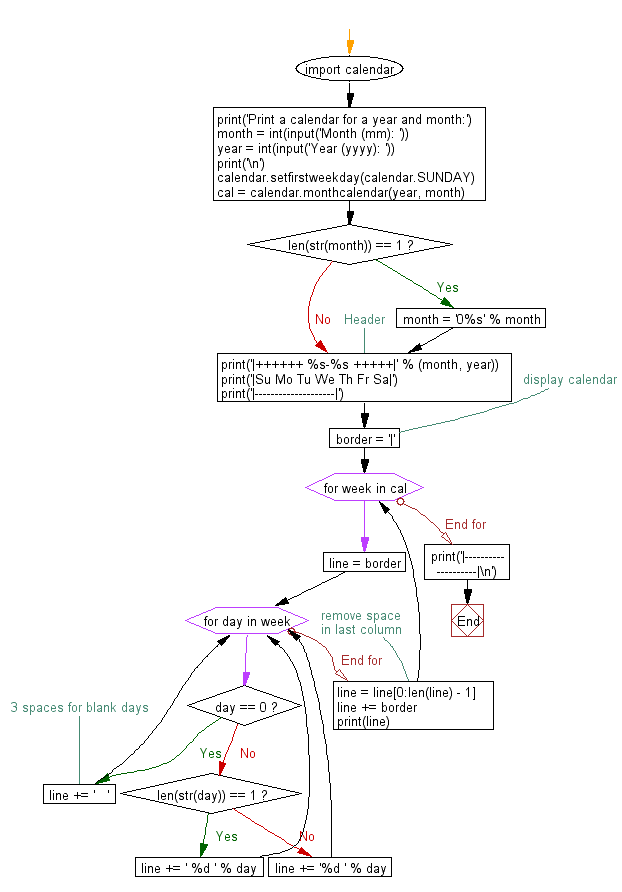﻿ Python: Display a simple, formatted calendar of a given year and month - w3resource

# Python: Display a simple, formatted calendar of a given year and month

## Python Datetime: Exercise-48 with Solution

Write a Python program to display a simple, formatted calendar of a given year and month.

Sample Solution:

Python Code:

``````import calendar
print('Print a calendar for a year and month:')
month = int(input('Month (mm): '))
year = int(input('Year (yyyy): '))
print('\n')

calendar.setfirstweekday(calendar.SUNDAY)
cal = calendar.monthcalendar(year, month)

if len(str(month)) == 1:
month = '0%s' % month

print('|++++++ %s-%s +++++|' % (month, year))
print('|Su Mo Tu We Th Fr Sa|')
print('|--------------------|')

# display calendar
border = '|'
for week in cal:
line = border

for day in week:
if day == 0:
# 3 spaces for blank days
line += '   '
elif len(str(day)) == 1:
line += ' %d ' % day
else:
line += '%d ' % day
# remove space in last column
line = line[0:len(line) - 1]
line += border
print(line)

print('|--------------------|\n')
```
```

Sample Output:

```Print a calendar for a year and month:
Month (mm): 05
Year (yyyy): 2017

|++++++ 05-2017 +++++|
|Su Mo Tu We Th Fr Sa|
|--------------------|
|    1  2  3  4  5  6|
| 7  8  9 10 11 12 13|
|14 15 16 17 18 19 20|
|21 22 23 24 25 26 27|
|28 29 30 31         |
|--------------------|
```

Flowchart:Python Code Editor: